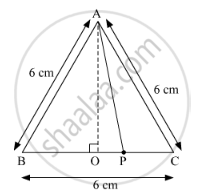Share

# ∆Abc is an Equilateral Triangle. Point P is on Base Bc Such that Pc = 1 3 Bc, If Ab = 6 Cm Find Ap. - Geometry

ConceptSimilarity in Right Angled Triangles

#### Question

∆ABC is an equilateral triangle. Point P is on base BC such that PC = $\frac{1}{3}$ BC, if AB = 6 cm find AP.

#### Solution∆ABC is an equilateral triangle.
It is given that,

$PC = \frac{1}{3}BC$
$\Rightarrow PC = \frac{1}{3} \times 6$
$\Rightarrow PC = 2 cm$
$\Rightarrow BP = 4 cm$

Since, ABC is an equilateral triangle, OA is the perpendicular bisector of BC.
∴ OC = 3 cm
⇒ OP = OC − PC
= 3 − 2
= 1              ...(1)

Now, According to Pythagoras theorem,
In ∆AOB,

${AB}^2 = {AO}^2 + {OB}^2$
$\Rightarrow \left( 6 \right)^2 = {AO}^2 + \left( 3 \right)^2$
$\Rightarrow 36 - 9 = {AO}^2$
$\Rightarrow {AO}^2 = 27$
$\Rightarrow AO = 3\sqrt{3} cm . . . \left( 2 \right)$

In ∆AOP,

${AP}^2 = {AO}^2 + {OP}^2$
$\Rightarrow {AP}^2 = \left( 3\sqrt{3} \right)^2 + \left( 1 \right)^2 \left( \text{From} \left( 1 \right) \text{and} \left( 2 \right) \right)$
$\Rightarrow {AP}^2 = 27 + 1$
$\Rightarrow {AP}^2 = 28$
$\Rightarrow AP = 2\sqrt{7} cm$

Hence, AP = 2$\sqrt{7}$ cm.

Is there an error in this question or solution?

#### Video TutorialsVIEW ALL 

Solution ∆Abc is an Equilateral Triangle. Point P is on Base Bc Such that Pc = 1 3 Bc, If Ab = 6 Cm Find Ap. Concept: Similarity in Right Angled Triangles.
S